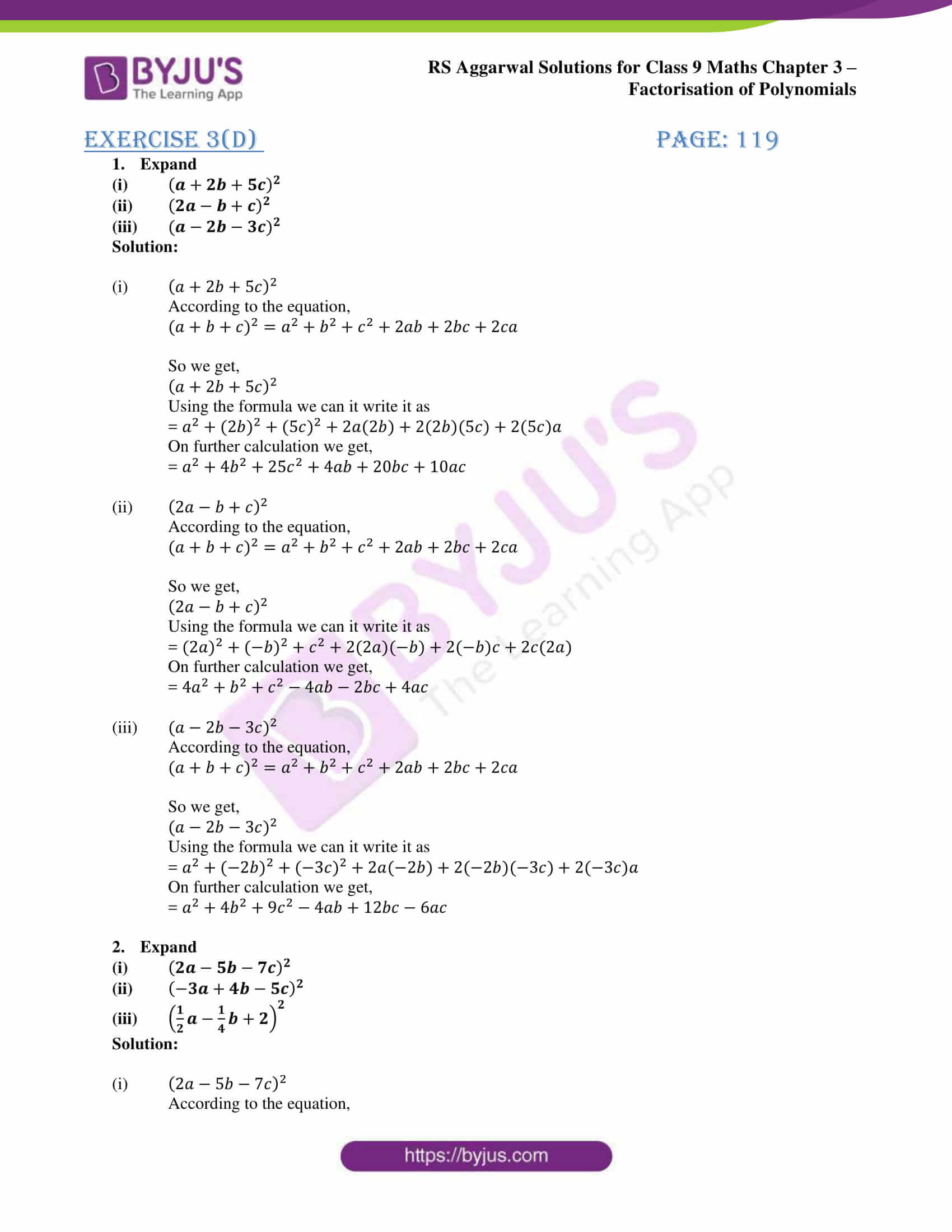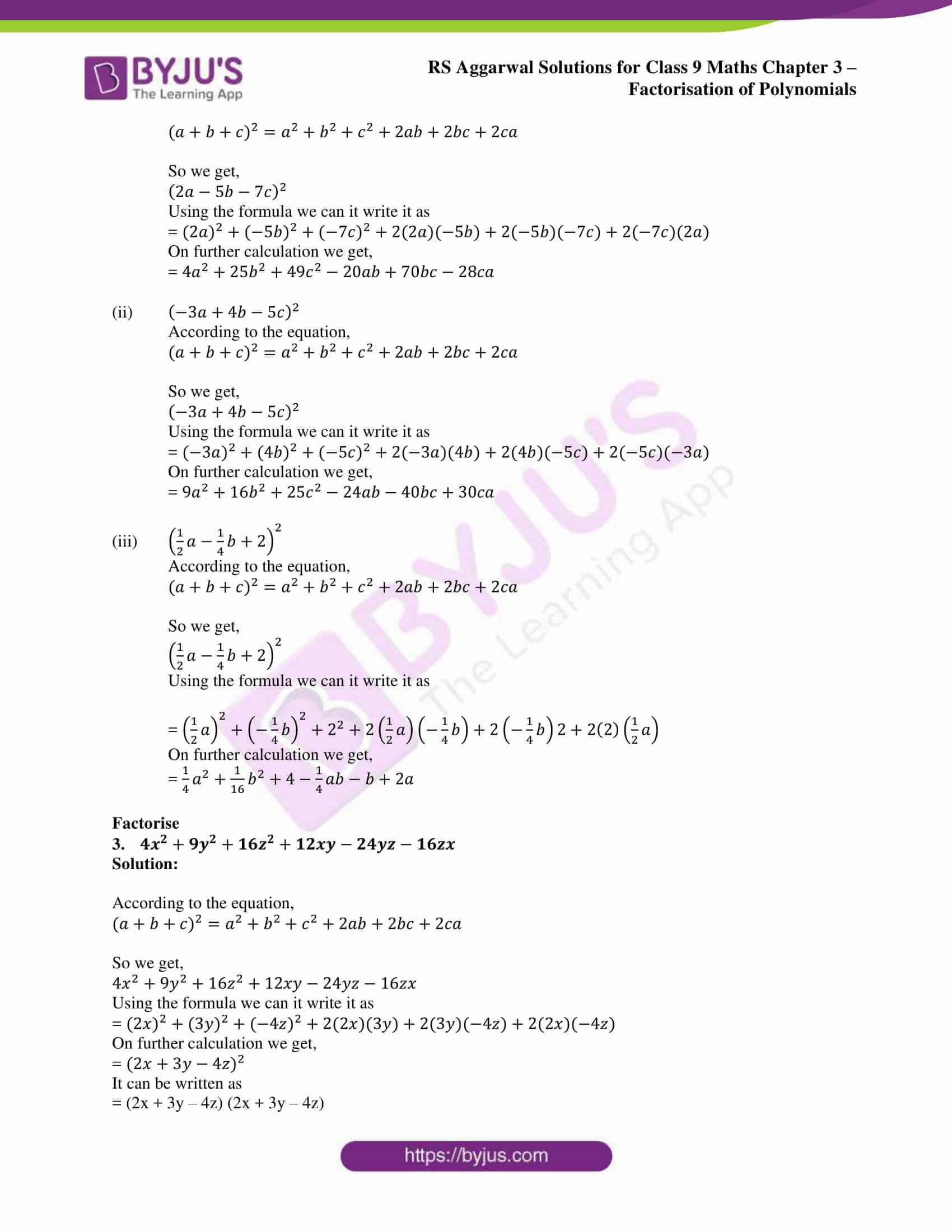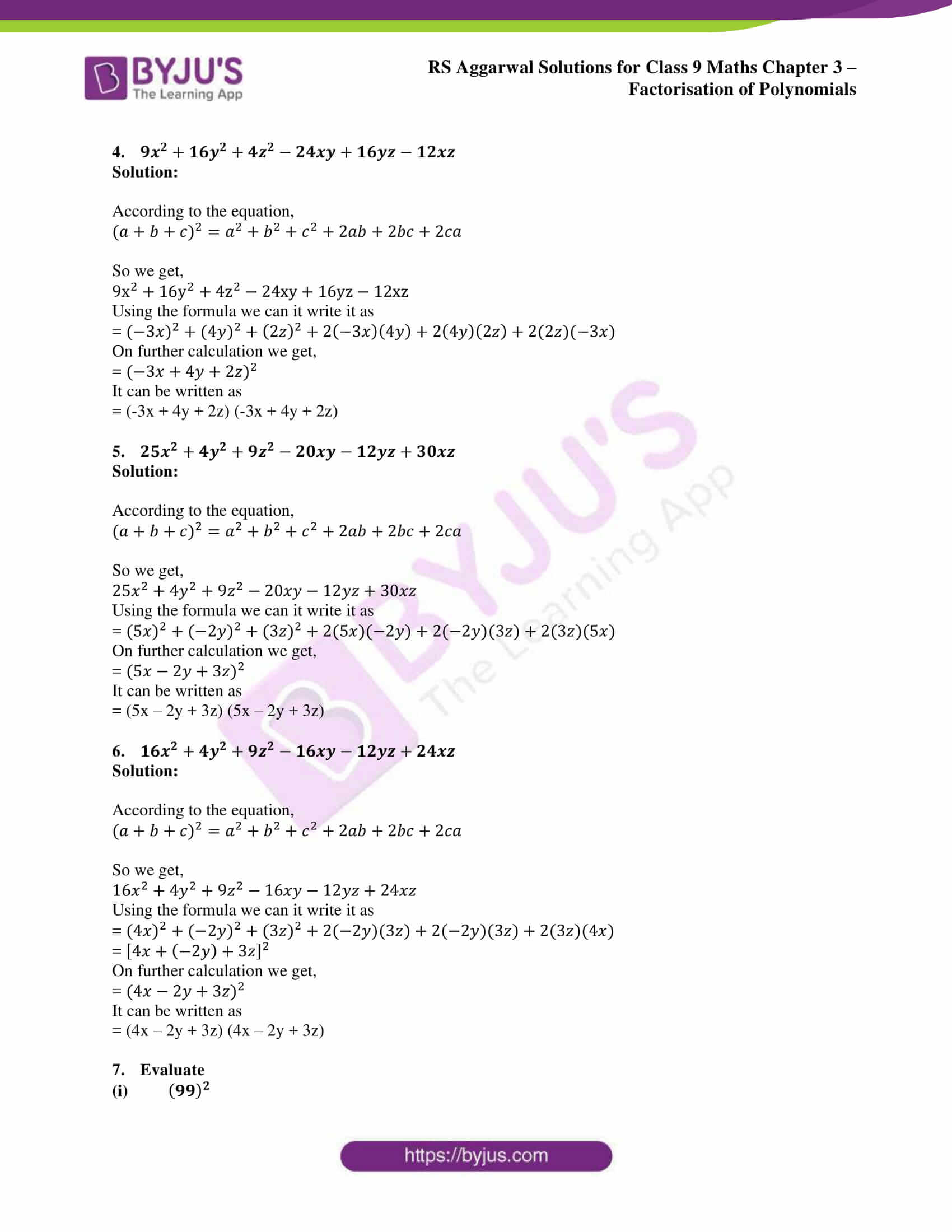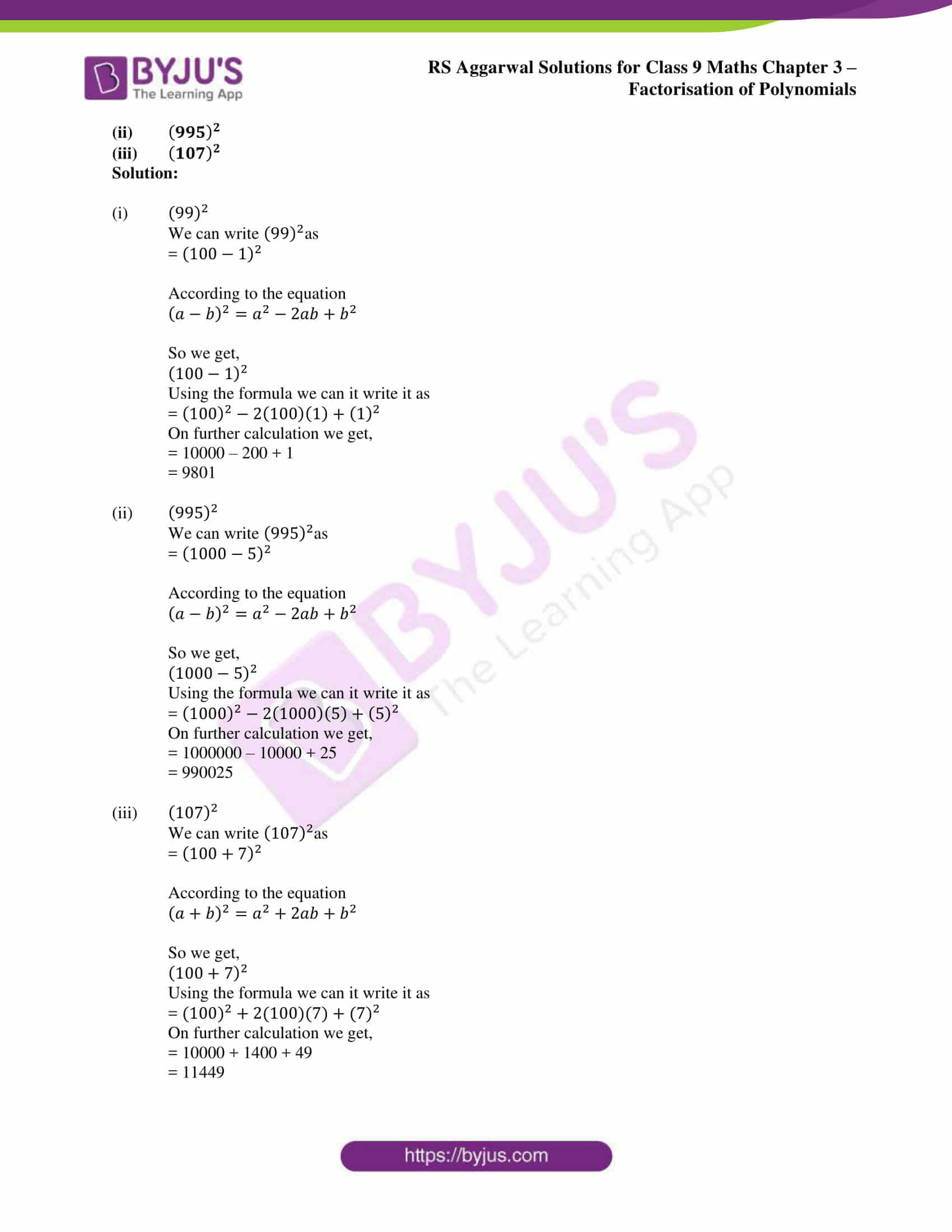# RS Aggarwal Solutions for Class 9 Chapter 3: Factorisation of Polynomials Exercise 3D

## RS Aggarwal Solutions for Class 9 Maths Exercise 3D PDF

RS Aggarwal Solutions are prepared by knowledgeable faculties with the aim of making Mathematics easier for the students. While solving the exercise wise problems many number of doubts arise in the minds of students, hence the solutions prepared are available in PDF format, which can be used as a reference. The solutions are mainly for the students of CBSE syllabus in order to help them clear the exams with a high score. Each and every problem is solved by keeping in mind the evaluation process for each step followed in the exams. RS Aggarwal Solutions for Class 9 Maths Chapter 3 Factorisation of Polynomials Exercise 3D are provided here.

## RS Aggarwal Solutions for Class 9 Chapter 3: Factorisation of Polynomials Exercise 3D Download PDF### Access other exercise solutions of class 9 Maths Chapter 3: Factorisation of Polynomials

Exercise 3A Solutions 34 Questions

Exercise 3B Solutions 40 Questions

Exercise 3C Solutions 66 Questions

Exercise 3E Solutions 10 Questions

Exercise 3F Solutions 38 Questions

Exercise 3G Solutions 25 Questions

### RS Aggarwal Solutions Class 9 Maths Chapter 3 – Factorisation of Polynomials Exercise 3D

RS Aggarwal Solutions Class 9 Maths Chapter 3 Factorisation of Polynomials Exercise 3D has a set of problems solved on the topic Square of a Trinomial which are made available in PDF format.

### Key features of RS Aggarwal Solutions for Class 9 Maths Chapter 3: Factorisation of Polynomials Exercise 3D

• RS Aggarwal Solutions for Class 9 Maths Chapter 3 helps students to solve and clarify doubts to the questions in Exercise 3D.
• PDF format of the solutions would mainly help students fetch more marks in the Class 9 exams.
• The solutions mainly follow CBSE guidelines so that the students perform well in the exams without fear.
• The problems which are solved are repeated questions from previous year question papers.